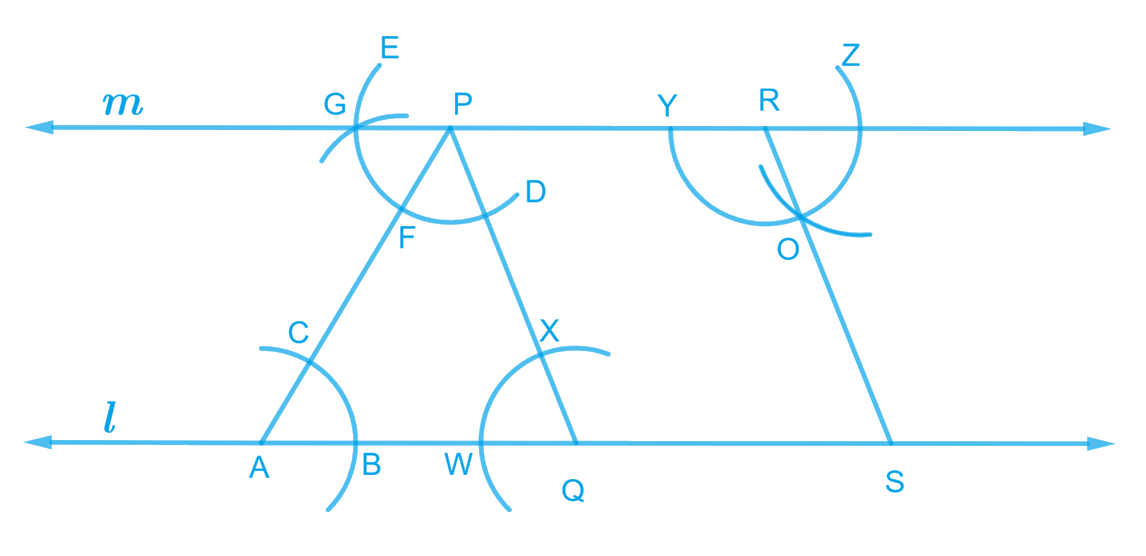# Ex.10.1 Q3 Practical Geometry Solution- NCERT Maths Class 7

Go back to  'Ex.10.1'

## Question

Let $$l$$ be a line and$$\,P\,$$be a point not on $$l$$. Through $$P ,$$ draw a line$$\,m\,$$parallel to $$l$$. Now join$$\,P\,$$to any point $$Q$$ on $$l$$. Choose any other point $$R$$ on$$\, m \,$$. Through $$\,R,\,$$ draw a line parallel to $$PQ$$. Let this meet $$l$$ at $$S.$$ What shape do the two sets of parallel lines enclose?

Video Solution
Practical Geometry
Ex 10.1 | Question 3

## Text Solution

What is known?

A line $$l$$ and a point $$P$$ not on $$l$$ and line m parallel to $$l$$..

What is unknown?

Shape formed by two sets of two parallel lines.

Reasoning:

As line $$l$$ is given and$$\,P\,$$is a point not on $$l$$. Through $$P,$$ draw a line$$\,m\,$$parallel to $$l$$. Now join$$\,P\,$$to any point $$Q$$ on $$l$$. Choose any other point $$R$$ on$$\, m \,$$. Through $$\,R,\,$$ draw a line parallel to $$PQ.$$ Let this meet $$l$$ at $$S.$$

Steps:Steps of construction –

1. Draw a line $$l$$, take a point $$A$$ on it. Take a point$$\,P\,$$not on $$l$$ and join $$A$$ to $$P.$$
2. Taking $$A$$ as centre and with a convenient radius draw an arc cutting $$l$$ at $$B$$ and $$AP$$ at $$C.$$
3. Taking$$\,P\,$$as the centre and with the same radius as before, draw an arc $$DE$$ to intersect $$AP$$ at $$F.$$
4. Adjust the compasses up to the length of $$BC.$$ Without changing the opening of compasses and taking $$F$$ as the centre, draw an arc to intersect the previous drawn arc $$DE$$ at point $$G.$$
5. Join$$\,P\,$$to any point $$G$$ to draw line$$\, m \,$$. Line$$\,m\,$$will be parallel to $$l$$.
6. Join$$\,P\,$$to any point $$Q$$ on line $$l$$. Choose another point $$R$$ on line$$\, m \,$$. Similarly, a line can be drawn through point $$R$$ and parallel to $$PQ.$$
7. Let it meet line $$l$$ at point $$S.$$

In quadrilateral $$PQSR$$, opposite lines are parallel to each other.

Thus, $$PQSR$$ is a parallelogram.

Learn from the best math teachers and top your exams

• Live one on one classroom and doubt clearing
• Practice worksheets in and after class for conceptual clarity
• Personalized curriculum to keep up with school